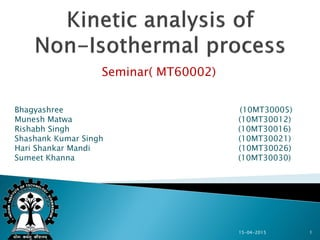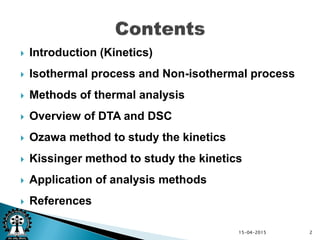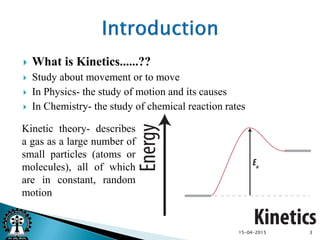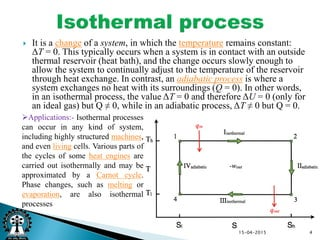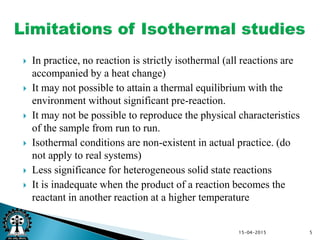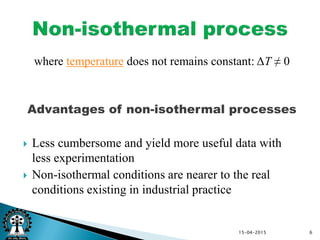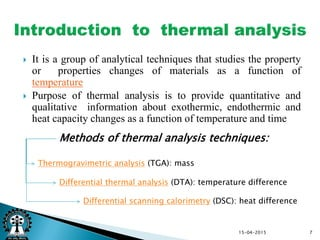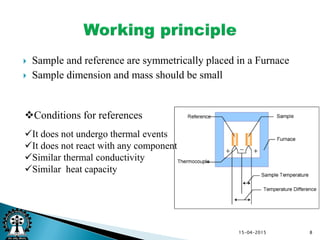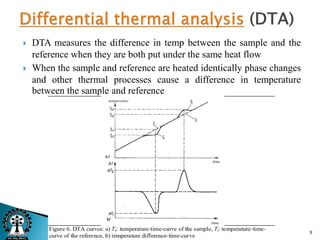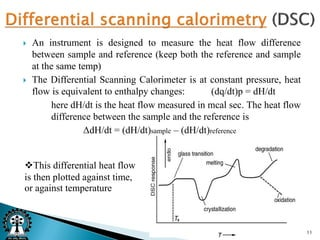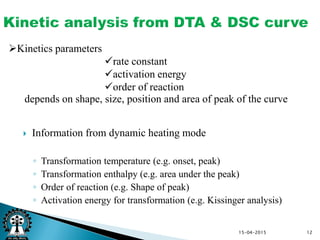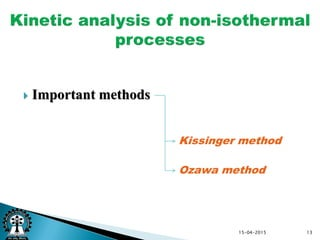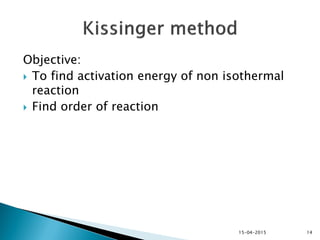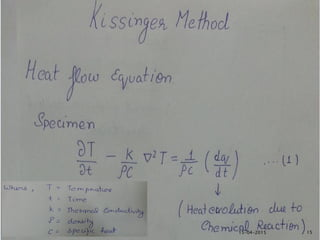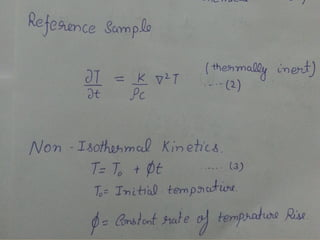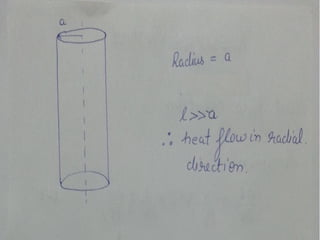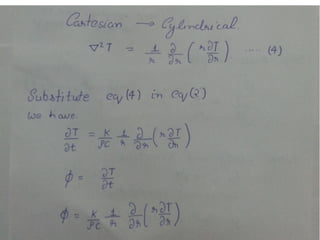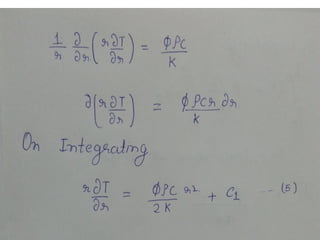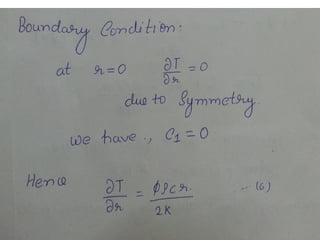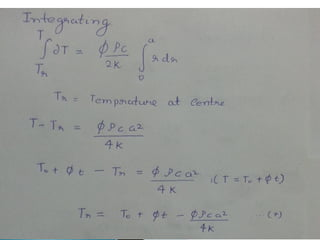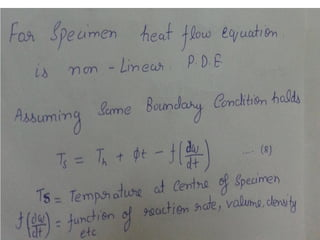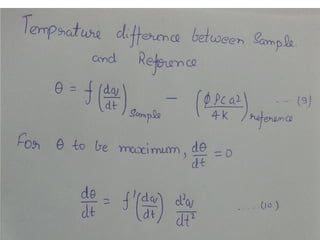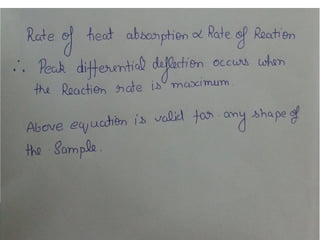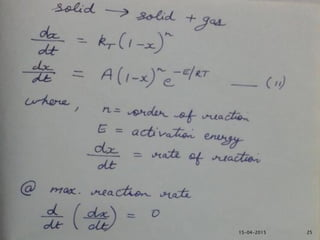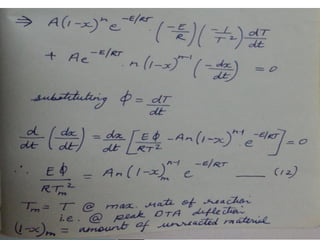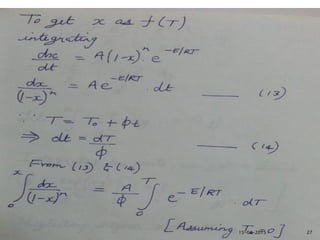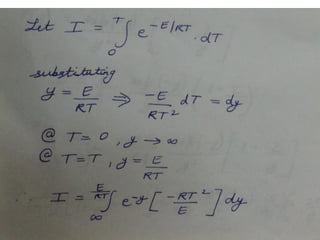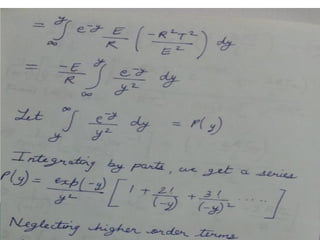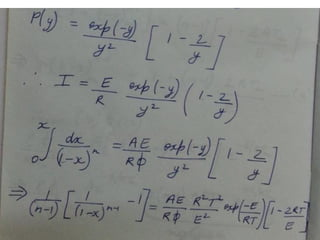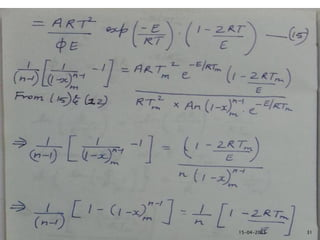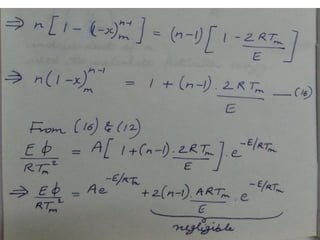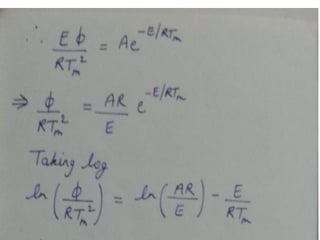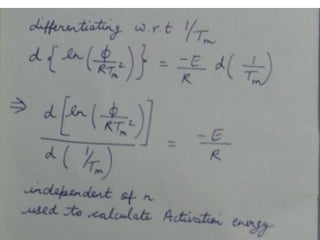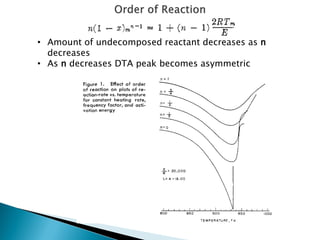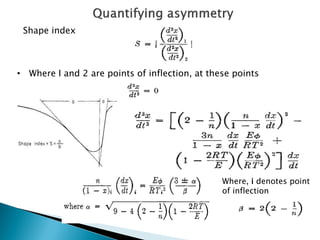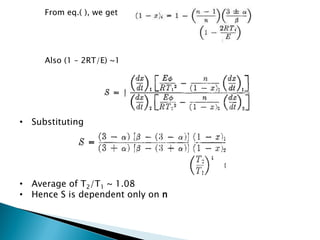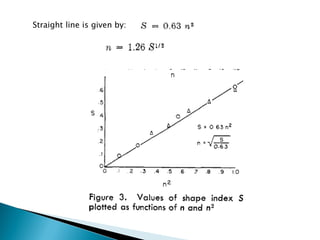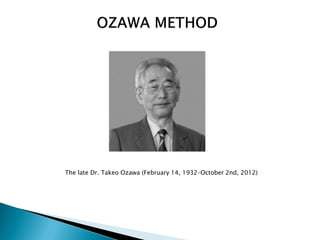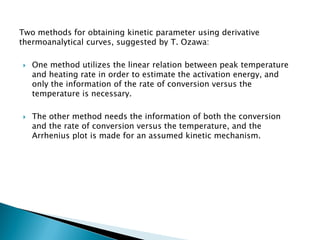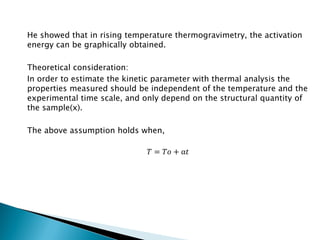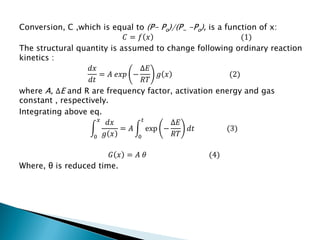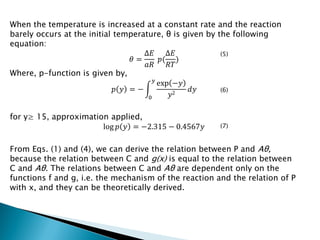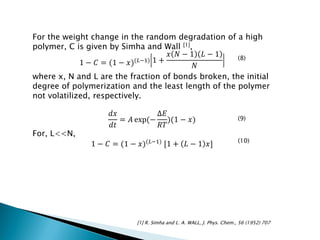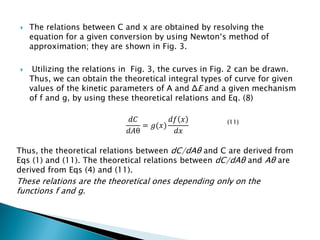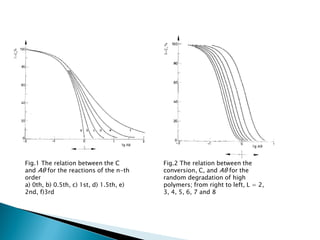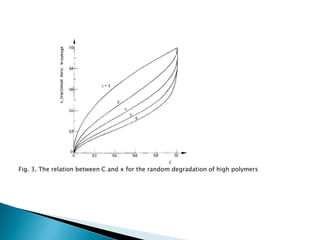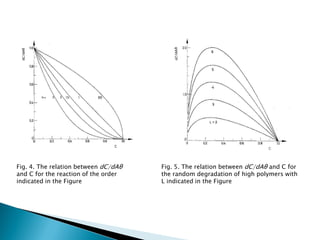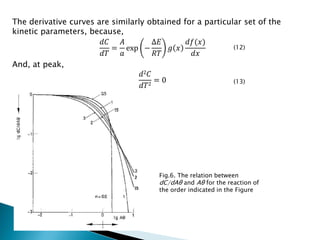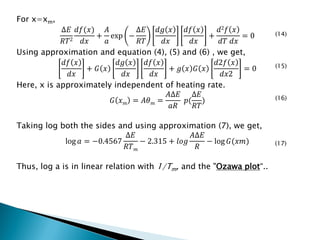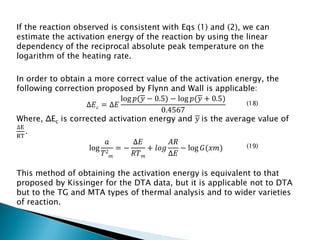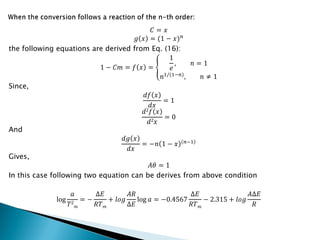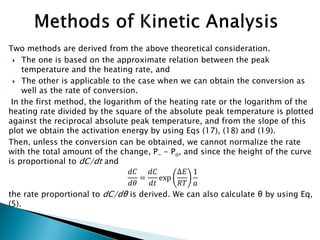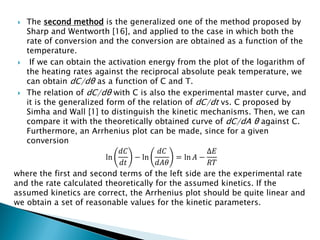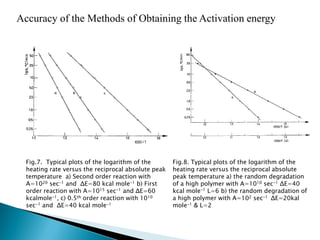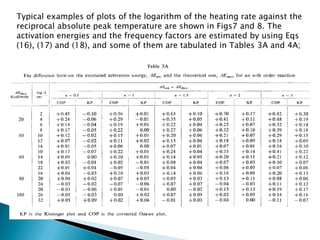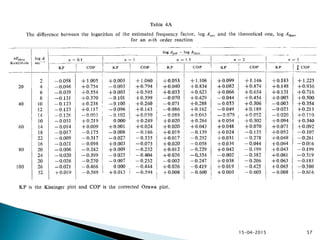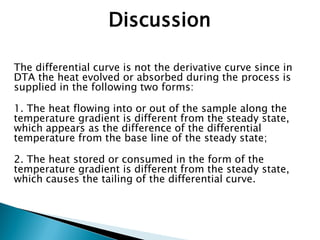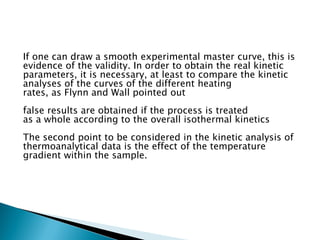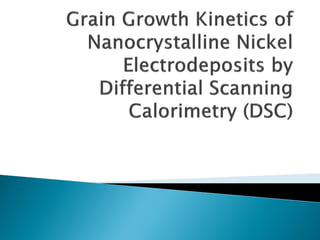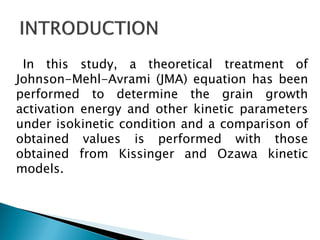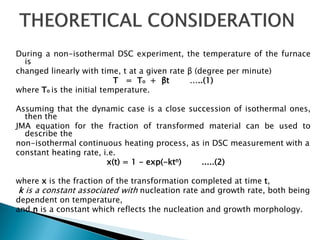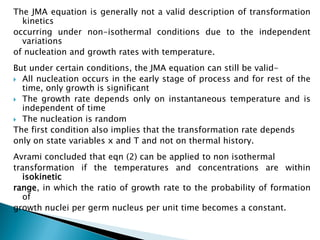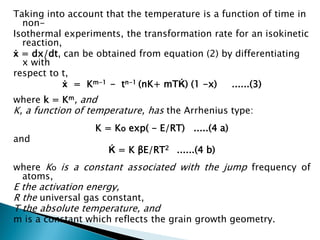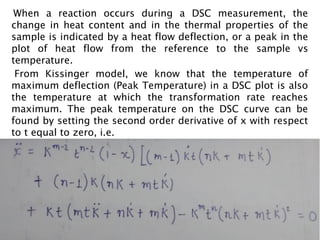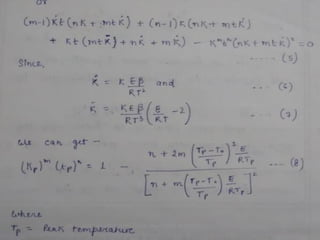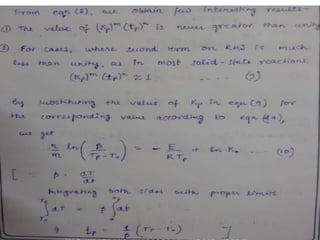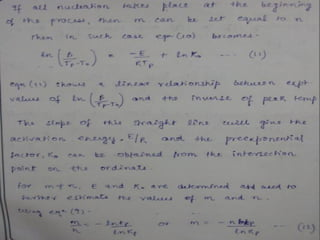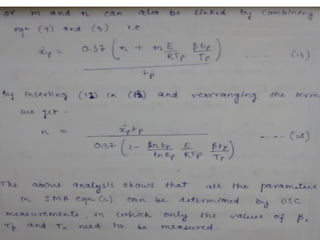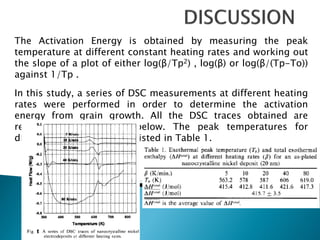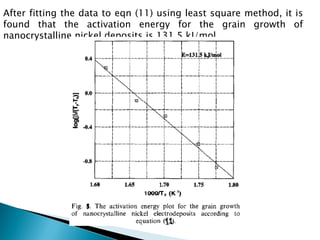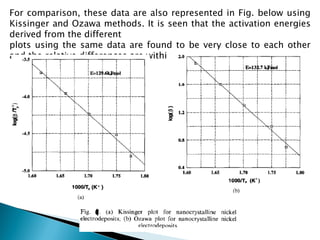1 de 73

### Non isothermal kinetics in phase transformations

1. Seminar( MT60002) Bhagyashree (10MT30005) Munesh Matwa (10MT30012) Rishabh Singh (10MT30016) Shashank Kumar Singh (10MT30021) Hari Shankar Mandi (10MT30026) Sumeet Khanna (10MT30030) 15-04-2015 1
2.  Introduction (Kinetics)  Isothermal process and Non-isothermal process  Methods of thermal analysis  Overview of DTA and DSC  Ozawa method to study the kinetics  Kissinger method to study the kinetics  Application of analysis methods  References 15-04-2015 2
3.  What is Kinetics......??  Study about movement or to move  In Physics- the study of motion and its causes  In Chemistry- the study of chemical reaction rates 15-04-2015 3 Kinetic theory- describes a gas as a large number of small particles (atoms or molecules), all of which are in constant, random motion
4.  It is a change of a system, in which the temperature remains constant: ΔT = 0. This typically occurs when a system is in contact with an outside thermal reservoir (heat bath), and the change occurs slowly enough to allow the system to continually adjust to the temperature of the reservoir through heat exchange. In contrast, an adiabatic process is where a system exchanges no heat with its surroundings (Q = 0). In other words, in an isothermal process, the value ΔT = 0 and therefore ΔU = 0 (only for an ideal gas) but Q ≠ 0, while in an adiabatic process, ΔT ≠ 0 but Q = 0. 15-04-2015 4 Applications:- Isothermal processes can occur in any kind of system, including highly structured machines, and even living cells. Various parts of the cycles of some heat engines are carried out isothermally and may be approximated by a Carnot cycle. Phase changes, such as melting or evaporation, are also isothermal processes
5.  In practice, no reaction is strictly isothermal (all reactions are accompanied by a heat change)  It may not possible to attain a thermal equilibrium with the environment without significant pre-reaction.  It may not be possible to reproduce the physical characteristics of the sample from run to run.  Isothermal conditions are non-existent in actual practice. (do not apply to real systems)  Less significance for heterogeneous solid state reactions  It is inadequate when the product of a reaction becomes the reactant in another reaction at a higher temperature 15-04-2015 5
6. where temperature does not remains constant: ΔT ≠ 0 Advantages of non-isothermal processes  Less cumbersome and yield more useful data with less experimentation  Non-isothermal conditions are nearer to the real conditions existing in industrial practice 15-04-2015 6
7.  It is a group of analytical techniques that studies the property or properties changes of materials as a function of temperature  Purpose of thermal analysis is to provide quantitative and qualitative information about exothermic, endothermic and heat capacity changes as a function of temperature and time 15-04-2015 7 Methods of thermal analysis techniques: Thermogravimetric analysis (TGA): mass Differential thermal analysis (DTA): temperature difference Differential scanning calorimetry (DSC): heat difference
8.  Sample and reference are symmetrically placed in a Furnace  Sample dimension and mass should be small 15-04-2015 8 Conditions for references It does not undergo thermal events It does not react with any component Similar thermal conductivity Similar heat capacity
9.  DTA measures the difference in temp between the sample and the reference when they are both put under the same heat flow  When the sample and reference are heated identically phase changes and other thermal processes cause a difference in temperature between the sample and reference 15-04-2015 9
10.  Changes in the sample which lead to the absorption or evolution of heat can be detected relative to the inert reference  This differential temperature is then plotted against time, or against temperature 15-04-2015 10
11.  An instrument is designed to measure the heat flow difference between sample and reference (keep both the reference and sample at the same temp)  The Differential Scanning Calorimeter is at constant pressure, heat flow is equivalent to enthalpy changes: (dq/dt)p = dH/dt here dH/dt is the heat flow measured in mcal sec. The heat flow difference between the sample and the reference is ΔdH/dt = (dH/dt)sample – (dH/dt)reference 15-04-2015 11 This differential heat flow is then plotted against time, or against temperature
12.  Information from dynamic heating mode ◦ Transformation temperature (e.g. onset, peak) ◦ Transformation enthalpy (e.g. area under the peak) ◦ Order of reaction (e.g. Shape of peak) ◦ Activation energy for transformation (e.g. Kissinger analysis) 15-04-2015 12 Kinetics parameters rate constant activation energy order of reaction depends on shape, size, position and area of peak of the curve
13.  Important methods 15-04-2015 13 Kissinger method Ozawa method
14. Objective:  To find activation energy of non isothermal reaction  Find order of reaction 15-04-2015 14
15. 15-04-2015 15
16. 15-04-2015 16
17. 15-04-2015 17
18. 15-04-2015 18
19. 15-04-2015 19
20. 15-04-2015 20
21. 15-04-2015 21
22. 15-04-2015 22
23. 15-04-2015 23
24. 15-04-2015 24
25. 15-04-2015 25
26. 15-04-2015 26
27. 15-04-2015 27
28. 15-04-2015 28
29. 15-04-2015 29
30. 15-04-2015 30
31. 15-04-2015 31
32. 15-04-2015 32
33. 15-04-2015 33
34. 15-04-2015 34
35. • Amount of undecomposed reactant decreases as n decreases • As n decreases DTA peak becomes asymmetric
36. Shape index • Where I and 2 are points of inflection, at these points Where, i denotes point of inflection
37. • Substituting • Average of T2/T1 ~ 1.08 • Hence S is dependent only on n From eq.( ), we get Also (1 – 2RT/E) ~1
38. Straight line is given by:
39. The late Dr. Takeo Ozawa (February 14, 1932–October 2nd, 2012)
40. Two methods for obtaining kinetic parameter using derivative thermoanalytical curves, suggested by T. Ozawa:  One method utilizes the linear relation between peak temperature and heating rate in order to estimate the activation energy, and only the information of the rate of conversion versus the temperature is necessary.  The other method needs the information of both the conversion and the rate of conversion versus the temperature, and the Arrhenius plot is made for an assumed kinetic mechanism.
41. He showed that in rising temperature thermogravimetry, the activation energy can be graphically obtained. Theoretical consideration: In order to estimate the kinetic parameter with thermal analysis the properties measured should be independent of the temperature and the experimental time scale, and only depend on the structural quantity of the sample(x). The above assumption holds when, 𝑇 = 𝑇𝑜 + α𝑡
42. Conversion, C ,which is equal to (P- Po)/(P~ -Po), is a function of x: 𝐶 = 𝑓 𝑥 (1) The structural quantity is assumed to change following ordinary reaction kinetics : 𝑑𝑥 𝑑𝑡 = 𝐴 𝑒𝑥𝑝 − Δ𝐸 𝑅𝑇 𝑔 𝑥 (2) where A, ΔE and R are frequency factor, activation energy and gas constant , respectively. Integrating above eq. 𝑑𝑥 𝑔 𝑥 = 𝐴 exp − ∆𝐸 𝑅𝑇 𝑑𝑡 (3) 𝑡 0 𝑥 0 𝐺 𝑥 = 𝐴 𝜃 (4) Where, θ is reduced time.
43. When the temperature is increased at a constant rate and the reaction barely occurs at the initial temperature, θ is given by the following equation: 𝜃 = ∆𝐸 𝑎𝑅 𝑝( ∆𝐸 𝑅𝑇 ) Where, p-function is given by, 𝑝 𝑦 = − exp −𝑦 𝑦2 𝑑𝑦 𝑦 0 for y≥ 15, approximation applied, log 𝑝 𝑦 = −2.315 − 0.4567𝑦 From Eqs. (1) and (4), we can derive the relation between P and Aθ, because the relation between C and g(x) is equal to the relation between C and Aθ. The relations between C and Aθ are dependent only on the functions f and g, i.e. the mechanism of the reaction and the relation of P with x, and they can be theoretically derived. (5) (6) (7)
44. For the weight change in the random degradation of a high polymer, C is given by Simha and Wall , 1 − 𝐶 = (1 − 𝑥)(𝐿−1) 1 + 𝑥 𝑁 − 1 𝐿 − 1 𝑁 where x, N and L are the fraction of bonds broken, the initial degree of polymerization and the least length of the polymer not volatilized, respectively. 𝑑𝑥 𝑑𝑡 = 𝐴 exp(− ∆𝐸 𝑅𝑇 )(1 − 𝑥) For, L<<N, 1 − 𝐶 = (1 − 𝑥)(𝐿−1) [1 + 𝐿 − 1 𝑥]  R. Simha and L. A. WALL, J. Phys. Chem., 56 (1952) 707 (8) (9) (10)
45.  The relations between C and x are obtained by resolving the equation for a given conversion by using Newton‘s method of approximation; they are shown in Fig. 3.  Utilizing the relations in Fig. 3, the curves in Fig. 2 can be drawn. Thus, we can obtain the theoretical integral types of curve for given values of the kinetic parameters of A and ΔE and a given mechanism of f and g, by using these theoretical relations and Eq. (8) 𝑑𝐶 𝑑𝐴θ = 𝑔(𝑥) 𝑑𝑓 𝑥 𝑑𝑥 Thus, the theoretical relations between dC/dAθ and C are derived from Eqs (1) and (11). The theoretical relations between dC/dAθ and Aθ are derived from Eqs (4) and (11). These relations are the theoretical ones depending only on the functions f and g. (11)
46. Fig.1 The relation between the C and Aθ for the reactions of the n-th order a) 0th, b) 0.5th, c) 1st, d) 1.5th, e) 2nd, f)3rd Fig.2 The relation between the conversion, C, and Aθ for the random degradation of high polymers; from right to left, L = 2, 3, 4, 5, 6, 7 and 8
47. Fig. 3. The relation between C and x for the random degradation of high polymers
48. Fig. 4. The relation between dC/dAθ and C for the reaction of the order indicated in the Figure Fig. 5. The relation between dC/dAθ and C for the random degradation of high polymers with L indicated in the Figure
49. The derivative curves are similarly obtained for a particular set of the kinetic parameters, because, 𝑑𝐶 𝑑𝑇 = 𝐴 𝑎 exp − ∆𝐸 𝑅𝑇 𝑔 𝑥 𝑑𝑓(𝑥) 𝑑𝑥 And, at peak, 𝑑2 𝐶 𝑑𝑇2 = 0 Fig.6. The relation between dC/dAθ and Aθ for the reaction of the order indicated in the Figure (12) (13)
50. For x=xm, ∆𝐸 𝑅𝑇2 𝑑𝑓(𝑥) 𝑑𝑥 + 𝐴 𝑎 exp − ∆𝐸 𝑅𝑇 𝑑𝑔 𝑥 𝑑𝑥 𝑑𝑓 𝑥 𝑑𝑥 + 𝑑2 𝑓 𝑥 𝑑𝑇 𝑑𝑥 = 0 Using approximation and equation (4), (5) and (6) , we get, 𝑑𝑓 𝑥 𝑑𝑥 + 𝐺 𝑥 𝑑𝑔 𝑥 𝑑𝑥 𝑑𝑓 𝑥 𝑑𝑥 + 𝑔 𝑥 𝐺 𝑥 𝑑2𝑓 𝑥 𝑑𝑥2 = 0 Here, x is approximately independent of heating rate. 𝐺 𝑥 𝑚 = 𝐴𝜃 𝑚 = 𝐴∆𝐸 𝑎𝑅 𝑝( ∆𝐸 𝑅𝑇 ) Taking log both the sides and using approximation (7), we get, log 𝑎 = −0.4567 ∆𝐸 𝑅𝑇 𝑚 − 2.315 + 𝑙𝑜𝑔 𝐴∆𝐸 𝑅 − log 𝐺(𝑥𝑚) Thus, log a is in linear relation with 1/Tm, and the "Ozawa plot“.. (14) (15) (16) (17)
51. If the reaction observed is consistent with Eqs (1) and (2), we can estimate the activation energy of the reaction by using the linear dependency of the reciprocal absolute peak temperature on the logarithm of the heating rate. In order to obtain a more correct value of the activation energy, the following correction proposed by Flynn and Wall is applicable: ∆𝐸 𝑐 = ∆𝐸 log 𝑝(𝑦 − 0.5) − log 𝑝(𝑦 + 0.5) 0.4567 Where, ΔEc is corrected activation energy and 𝑦 is the average value of ∆E RT . log 𝑎 𝑇2 𝑚 = − ∆𝐸 𝑅𝑇 𝑚 + 𝑙𝑜𝑔 𝐴𝑅 ∆𝐸 − log 𝐺(𝑥𝑚) This method of obtaining the activation energy is equivalent to that proposed by Kissinger for the DTA data, but it is applicable not to DTA but to the TG and MTA types of thermal analysis and to wider varieties of reaction. (18) (19)
52. 𝐶 = 𝑥 𝑔 𝑥 = (1 − 𝑥) 𝑛 the following equations are derived from Eq. (16): 1 − 𝐶𝑚 = 𝑓 𝑥 = 1 𝑒 , 𝑛 = 1 𝑛1/(1−𝑛) , 𝑛 ≠ 1 Since, 𝑑𝑓 𝑥 𝑑𝑥 = 1 𝑑2 𝑓 𝑥 𝑑2 𝑥 = 0 And 𝑑𝑔 𝑥 𝑑𝑥 = −𝑛 1 − 𝑥 𝑛−1 Gives, 𝐴𝜃 = 1 In this case following two equation can be derives from above condition log 𝑎 𝑇2 𝑚 = − ∆𝐸 𝑅𝑇 𝑚 + 𝑙𝑜𝑔 𝐴𝑅 ∆𝐸 log 𝑎 = −0.4567 ∆𝐸 𝑅𝑇 𝑚 − 2.315 + 𝑙𝑜𝑔 𝐴∆𝐸 𝑅
53. Two methods are derived from the above theoretical consideration.  The one is based on the approximate relation between the peak temperature and the heating rate, and  The other is applicable to the case when we can obtain the conversion as well as the rate of conversion. In the first method, the logarithm of the heating rate or the logarithm of the heating rate divided by the square of the absolute peak temperature is plotted against the reciprocal absolute peak temperature, and from the slope of this plot we obtain the activation energy by using Eqs (17), (18) and (19). Then, unless the conversion can be obtained, we cannot normalize the rate with the total amount of the change, P~ - Po, and since the height of the curve is proportional to dC/dt and 𝑑𝐶 𝑑𝜃 = 𝑑𝐶 𝑑𝑡 exp ∆𝐸 𝑅𝑇 1 𝑎 the rate proportional to dC/dθ is derived. We can also calculate θ by using Eq, (5).
54.  The second method is the generalized one of the method proposed by Sharp and Wentworth , and applied to the case in which both the rate of conversion and the conversion are obtained as a function of the temperature.  If we can obtain the activation energy from the plot of the logarithm of the heating rates against the reciprocal absolute peak temperature, we can obtain dC/dθ as a function of C and T.  The relation of dC/dθ with C is also the experimental master curve, and it is the generalized form of the relation of dC/dt vs. C proposed by Simha and Wall  to distinguish the kinetic mechanisms. Then, we can compare it with the theoretically obtained curve of dC/dA θ against C. Furthermore, an Arrhenius plot can be made, since for a given conversion ln 𝑑𝐶 𝑑𝑡 − ln 𝑑𝐶 𝑑𝐴𝜃 = ln 𝐴 − ∆𝐸 𝑅𝑇 where the first and second terms of the left side are the experimental rate and the rate calculated theoretically for the assumed kinetics. If the assumed kinetics are correct, the Arrhenius plot should be quite linear and we obtain a set of reasonable values for the kinetic parameters.
55. Fig.8. Typical plots of the logarithm of the heating rate versus the reciprocal absolute peak temperature a) the random degradation of a high polymer with A=1010 sec-1 ΔE=40 kcal mole-1 L=6 b) the random degradation of a high polymer with A=102 sec-1 ΔE=20kal mole-1 & L=2 Accuracy of the Methods of Obtaining the Activation energy Fig.7. Typical plots of the logarithm of the heating rate versus the reciprocal absolute peak temperature a) Second order reaction with A=1020 sec-1 and ΔE=80 kcal mole-1 b) First order reaction with A=1015 sec-1 and ΔE=60 kcalmole-1, c) 0.5th order reaction with 1010 sec-1 and ΔE=40 kcal mole-1
56. Typical examples of plots of the logarithm of the heating rate against the reciprocal absolute peak temperature are shown in Figs7 and 8. The activation energies and the frequency factors are estimated by using Eqs (16), (17) and (18), and some of them are tabulated in Tables 3A and 4A;
57. 15-04-2015 57
58. The differential curve is not the derivative curve since in DTA the heat evolved or absorbed during the process is supplied in the following two forms: 1. The heat flowing into or out of the sample along the temperature gradient is different from the steady state, which appears as the difference of the differential temperature from the base line of the steady state; 2. The heat stored or consumed in the form of the temperature gradient is different from the steady state, which causes the tailing of the differential curve. Discussion
59. If one can draw a smooth experimental master curve, this is evidence of the validity. In order to obtain the real kinetic parameters, it is necessary, at least to compare the kinetic analyses of the curves of the different heating rates, as Flynn and Wall pointed out false results are obtained if the process is treated as a whole according to the overall isothermal kinetics The second point to be considered in the kinetic analysis of thermoanalytical data is the effect of the temperature gradient within the sample.
60. In this study, a theoretical treatment of Johnson-Mehl-Avrami (JMA) equation has been performed to determine the grain growth activation energy and other kinetic parameters under isokinetic condition and a comparison of obtained values is performed with those obtained from Kissinger and Ozawa kinetic models.
61. During a non-isothermal DSC experiment, the temperature of the furnace is changed linearly with time, t at a given rate β (degree per minute) T = To + βt …..(1) where To is the initial temperature. Assuming that the dynamic case is a close succession of isothermal ones, then the JMA equation for the fraction of transformed material can be used to describe the non-isothermal continuous heating process, as in DSC measurement with a constant heating rate, i.e. x(t) = 1 - exp(-ktn) .....(2) where x is the fraction of the transformation completed at time t, k is a constant associated with nucleation rate and growth rate, both being dependent on temperature, and n is a constant which reflects the nucleation and growth morphology.
62. The JMA equation is generally not a valid description of transformation kinetics occurring under non-isothermal conditions due to the independent variations of nucleation and growth rates with temperature. But under certain conditions, the JMA equation can still be valid-  All nucleation occurs in the early stage of process and for rest of the time, only growth is significant  The growth rate depends only on instantaneous temperature and is independent of time  The nucleation is random The first condition also implies that the transformation rate depends only on state variables x and T and not on thermal history. Avrami concluded that eqn (2) can be applied to non isothermal transformation if the temperatures and concentrations are within isokinetic range, in which the ratio of growth rate to the probability of formation of growth nuclei per germ nucleus per unit time becomes a constant.
63. Taking into account that the temperature is a function of time in non- Isothermal experiments, the transformation rate for an isokinetic reaction, ẋ = dx/dt, can be obtained from equation (2) by differentiating x with respect to t, ẋ = Km-1 - tn-1 (nK+ mTḰ) (1 -x) ......(3) where k = Km, and K, a function of temperature, has the Arrhenius type: K = Ko exp( - E/RT) .....(4 a) and Ḱ = K βE/RT2 ......(4 b) where Ko is a constant associated with the jump frequency of atoms, E the activation energy, R the universal gas constant, T the absolute temperature, and m is a constant which reflects the grain growth geometry.
64. When a reaction occurs during a DSC measurement, the change in heat content and in the thermal properties of the sample is indicated by a heat flow deflection, or a peak in the plot of heat flow from the reference to the sample vs temperature. From Kissinger model, we know that the temperature of maximum deflection (Peak Temperature) in a DSC plot is also the temperature at which the transformation rate reaches maximum. The peak temperature on the DSC curve can be found by setting the second order derivative of x with respect to t equal to zero, i.e.
65. The Activation Energy is obtained by measuring the peak temperature at different constant heating rates and working out the slope of a plot of either log(β/Tp2) , log(β) or log(β/(Tp-To)) against 1/Tp . In this study, a series of DSC measurements at different heating rates were performed in order to determine the activation energy from grain growth. All the DSC traces obtained are represented in the Fig below. The peak temperatures for different heating rates are listed in Table 1.
66. After fitting the data to eqn (11) using least square method, it is found that the activation energy for the grain growth of nanocrystalline nickel deposits is 131.5 kJ/mol.
67. For comparison, these data are also represented in Fig. below using Kissinger and Ozawa methods. It is seen that the activation energies derived from the different plots using the same data are found to be very close to each other and the relative differences are within 5%.
68. 15-04-2015 73 THANK YOU